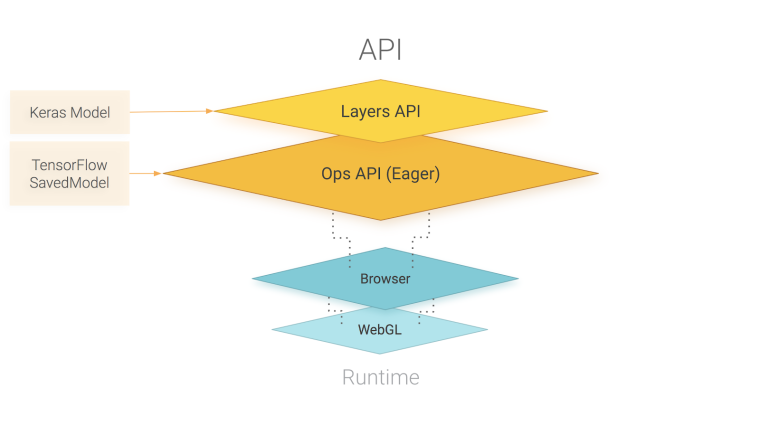TensorFlow.js，这是一个开源库，可以使用JavaScript和高级图层 API 在浏览器中定义，训练和运行完整的机器学习模型。如果您是ML的新手，那么TensorFlow.js是开始学习的好方法。或者，如果您是JavaScript新手的ML开发人员，请继续阅读以了解更多关于浏览器内ML的新机会。下面开始介绍它。

### 怎么使用起来

• 您可以导入现有的预先训练的模型进行推理。如果您有一个以前曾经脱机训练过的现有TensorFlow或Keras模型，则可以将其转换为TensorFlow.js格式，并将其加载到浏览器中进行预测。
• 您可以重新训练导入的模型。您可以使用传输学习来增强现有模型，使用名为“图像重新训练”的技术，使用浏览器中收集的少量数据进行离线培训。这是快速训练精确模型的一种方法，只使用少量数据。
• 直接在浏览器中创建模型。您还可以使用TensorFlow.js完全在浏览器中使用Javascript和高级图层API定义，训练和运行模型。

### 代码工作过程

``````import * as tf from ‘@tensorflow/tfjs’;
const model = tf.sequential();
model.compile({loss: ‘categoricalCrossentropy’, optimizer: ‘sgd’});``````

``````await model.fit(
xData, yData, {
batchSize: batchSize,
epochs: epochs
});``````

``````// Get measurements for a new flower to generate a prediction
// The first argument is the data, and the second is the shape.
const inputData = tf.tensor2d([[4.8, 3.0, 1.4, 0.1]], [1, 4]);

// Get the highest confidence prediction from our model
const result = model.predict(inputData);
const winner = irisClasses[result.argMax().dataSync()];

// Display the winner
console.log(winner);``````

### 架构原理TensorFlow.js 主要是由WebGL提供能力支持，并且 TensorFlow.js 提供了一个用于定义模型的高层 API，以及用于线性代数和自动微分的低级 API。TensorFlow.js支持导入TensorFlow SavedModels 和 Keras 模型。

### FAQ

TensorFlow.js 现在支持 Node.js 吗？

TensorFlow.js 与 TensorFlow 的关系？
TensorFlow.js 的 API 跟 TensorFlow Python API 是类似的，当然目前还未全部支持，后面会跟 Python API 一样，提供相同的 API

TensorFlow.js 和 deeplearn.js的区别？
TensorFlow.js 是由从 deeplearn.js 发展而来，围绕深度学习提供一整套 JavaScript 的工具的系统。deeplearn.js 可以被认为是 TensorFlow.js 的 Core 。

Responses